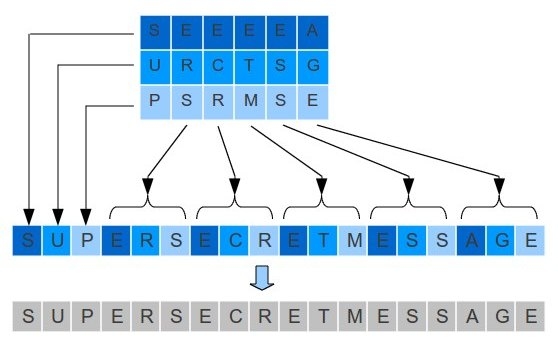## Introduction to Network Security – Part 11

NOTIFICATION: These examples are provided for educational purposes. The use of this code and/or information is under your own responsibility and risk. The information and/or code is given ‘as is’. I do not take responsibilities of how they are used. You are welcome to point out any mistakes in my posting and/or leave a comment.

Key Distribution Using Public-Key Cryptography

In the previous post, introduction to network security – part 10, we saw three main methods of public-key:

1. Public announcement,
2. Public-key authority, and
3. Public-key certificates

These methods can be used for encryption and decryption of messages (secrecy) and/or authentication.

These methods the disadvantage of being slow; therefore, its common to use symmetric-key encryption for secrecy and distribute using public-key encryption session keys. In this way we use the advantage of the speed of symmetric-key encryption and the security of public-key encryption.

Simple Key Distribution

In 1979,  Ralph C. Merkle created his thesis entitled “Secrecy, authentication and public key systems” which let him receive his Ph. D. in Electrical Engineering at Stanford University <http://en.wikipedia.org/wiki/Ralph_Merkle>.

For a key distribution, Merkle proposed:

1. User A will generate a new temporaty public key pair, PUa
2. User A send the public key, PUa, to user B together with its identity, IDa
PUa, IDa
3. User B generate the session key K.
4. User B uses the public key, PUa, supplied by user A to encrypt the session key K. Then user B send the encrypted session to user A
5. User A decrypt the message to obtain the session key K.
6. User A discards the public key PUa
7. User B discards user A’s public key, PUa.
8. After the exchange of information is complete, user A and B discard the session key K.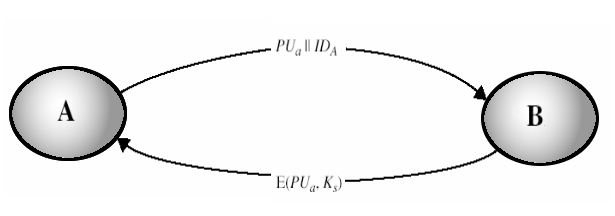The Man-In-The-Middle Attack

This type of key distribution have a disadvantage.  Lets assume that we have an attacker that gets in the middle of the communication in a way that this attacker can intercept the messages and then replay this message, modify this message, or send another different message.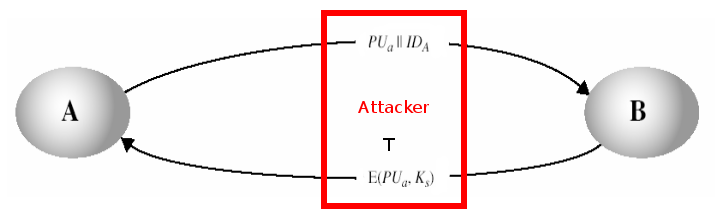Lets analyse this problem:

1. User A send a message to user B which holds the public key PUa, and user A’s identifier IDa
2. The attacker T intercept this message and create its own pair keys, public key PUt and private key PRt:
{PUt, PRt}

3. The attacker T send to user B, its own public key PUt together with the user A’s identification IDa :
PUt||IDa
4. User B generate a session key Ks. Then user B send this session key Ks encrypted using the public-key PUt that he received thinking that it came from user A.
Ciphertext = E(PUt, Ks)
5. The attacker T intercept the message obtaining the session key Ks by decrypting the message with his private key PRt.
Ks = D(PRt, Ciphertext) = D(PRt, E(PUt, Ks))
6. Then attacker T send the key session Ks to the user A using user A’s public key PUa
7. Without user A and B knowing, the attacket T obtained the session Ks successfully.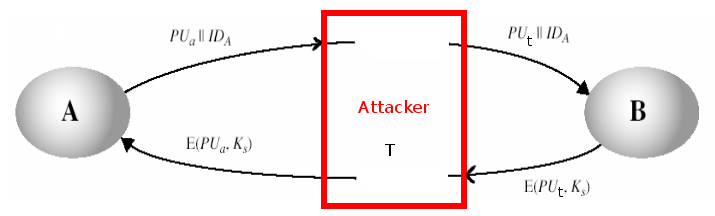Solution to The Man-In-The-Middle Attack

1. The process begins with user A. User A encrypt the message containing the user A identification IDa plus a nonce N1 using the user B’s public key PUb
2. User B generate a new nonce N2 and encrypts the message containing user A’s nonce N1 plus a new nonce N2 using the user A’s public key.
3. Since user B is the only one that could decrypted the first message coming from user A plus the new message send from user B to user A will contain the nonce N1 (given by  user A in the first message), user A will know the new message is coming from user B and not an attacker.
4. User A will encrypt nonce N2 using the public key PUb of user B. Then user A will send then encrypted nonce N2 to user B. In this way, since nonce N2 was generated by user B, when user B find nonce N2, user B will known the message came from user A.
5. User A generate a secret key Ks. User A will encrypt first the secret key Ks using the private key PUa of user A which would provide authentication, and then it will encrypt the output of the encryption with the public key PUb of user B to produce a new ciphertext M which provide confidentiality.
6. User B decrypt the ciphertext M by decrypting the ciphertext M using the private key PUb of userB, and the result will be decrypted again using the public key PUa of user A. In this way the secret key Ks is obtained.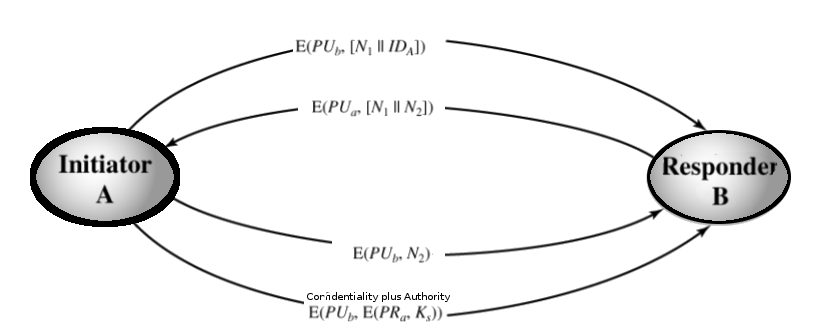Hybrid Key Distribution

Public key encryption is an algorithm that require a lot of processing. In a system that require to distribute session keys thought many users and require a frequently change of session keys, the public key encryption can slow the performance of the system as the load on the system keep increasing. One solution to this problem is to use an hybrid of different key distribution.

In an hybrid key distribution, the key distribution center (KDC) will be in charge of distributing a master key MK to each user of the system plus perform the distribution of session keys. Before these session keys are distributed, they will be encrypted by using the master key MK. Also, the master key is encrypted using a public key encryption. Since the master key only update in few occasions then the load of the system is reduced.

## Introduction to Network Security – Part 10

NOTIFICATION: These examples are provided for educational purposes. The use of this code and/or information is under your own responsibility and risk. The information and/or code is given ‘as is’. I do not take responsibilities of how they are used. You are welcome to point out any mistakes in my posting and/or leave a comment.

RSA Algorithm

RSA is an algorithm for public-key cryptography. The signals R.S.A. come from the last name of Ron Rivest, Adi Shamir, and Leonard Adleman who where the first to describe this algorithm. This algorithm is famous for being the first suitable algorithm for signing as well as encryption.

RSA algorithm allow to choose which key should be use for encryption and decryption.

1. Public key for encryption, private key for decryption or,
2. Private key for encryption, public key for decryption.

Generate the Pair Key (Public and Private Key)

1. Choose two random prime numbers p and q.
p = 17
q = 11

For better security, you can use the Primality Test to obtain to obtain these two random prime number. They should be of similar bit-length.
2. Compute n = p*q in which n is the modulus used for both the private and public keys.
n = p * q = 17 * 11 = 187
3. Compute Euler Totient Function ø(n)
ø(n) = ø(187) = (p – 1) * (q – 1) = 16 * 10 = 160
4. Select a public key exponent e number where 1 < e < ø(n) and gcd(e, ø(n)) = 1
If we choose e = 7 then gcd(e, ø(n)) = gcd(7, 160) = 1
5. Determine the multiplicative inverse d: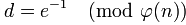1. d must be less than ø(n): d < 160
2. if d * e mod ø(n) = d * 7 mod ø(187) = d * 7 mod 160 = 1 then
3. let d = 23 in this way d * e = 23 * 7 = 161 = (160 + 1)
d * 7 mod 160 = 23 * 7 mod 160 = 1
6. The public key will be:
PU = {e, n} = {7, 187}
7. The private key will be:
PR = {d, n} = {23, 187}

Encryption

1. Sender must obtain the public key PU = {e, n} to the recipient, where PU is the public key, n for modulus, and e for public exponent (also known as public encryption).
PU = {e, n} = {7, 187}
2. The message M (also known as the plaintext) must be turn into an integer m by using a padding scheme (an reversible protocol) in which 0 < m < n.
Lets assume the message is m = 88 where 0 < m < n so 0 < 88 < 187.
3. Then the sender must compute the ciphertext.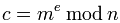Where c is the ciphertext, m is the integer message , e is the public exponent, and n i for modulus.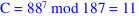Decryption

1. The recipient must use the private key to decrypt the ciphertext PR = {d, n} where PR is the private key, d is the private key exponent, n for modulus.
PR = {d, n} = {23, 187}
2. Compute the message.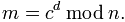Where m is the integer message, c is the ciphertext, n for modulus.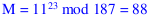3. Then turn back the original message M by using  integer message m with the reverse padding scheme.

Encryption / Decryption ExampleAlgorithm Requirements

1.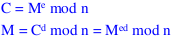2. There should be able to find values for e, d, and n so for all values of m where 0 < M < n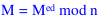3.andshould be easy to calculate for all valus of m where 0 < m < n.
4. It should be very hard for an attacker to determine d given e and n

Possible Attacks to RSA

1. Brute Attack
2. Mathematical attacks
1. Determine d directly
2. Determine the Euler Totient Function ø(n) without using the prime numbers p and q
3. Factorising n into the correct prime factors p and q

Key Distribution

One of the important aspects is how to distribute the keys between the sender and the receiver. For example, one way is to use the public-key encryption to distribute the keys.

For doing that there are three different methods of distributions that can be used:

1. Public announcement,
2. Public-key authority, and
3. Public-key certificates

Public Annoucement

One way to distribute the public keys is having the sender to distribute the public key to the recipient; however, this have the disadvantage that an attacker could create a key claiming to be the sender. This disadvantage is known as forgery.

A solution is to create a public-key autority.

Public Key Authority

A public key authority is a central authority that maintain a dynamic directory of public keys for all the users. Example: {name, public-key}

1. In a secure way (in person), each user register a public key in this directory authority.
2. It is required that the user known the public key for the directory.
3. Only the authority known the corresponding private key
4. Users interact with the directory in order to obtain the public key securely

Steps:

1. User A send  a timestamped message to the public key authority.
This message contain a request for the public key of user B.
2. The public-key authority responds to user A returning an encrypted message using it’s private key. This message contains:
1. The original request so it can be use to match with the request
2. The original timestamp so it can be determined if the message is not from the public-key authority.
3. The public key of user B.
3. User A store the public-key of user B and use this public-key to encrypt a message that will contain the identity of user A plus a “nonce N1”. This message will be deliver to user B.
4. User B send  a timestamped message to the public key authority.
This message contain a request for the public key of user A.
5. The public-key authority responds to user B returning an encrypted message using it’s private key. This message contains:
1. The original request so it can be use to match with the request
2. The original timestamp so it can be determined if the message is not from the public-key authority.
3. The public key of user A.
6. User B encrypt a message using the public-key of user A and send this encrypted message to user A.
This encrypted message have:

1. User A’s nonce
2. A nonce genereated by User B
7. User A encrypt a message using the public-key of User B and send this encrypted message to user B.
This encrypted message holds:

1. the  nonce N2 of user A

(This will ensure user B that the encrypted message is coming from user A).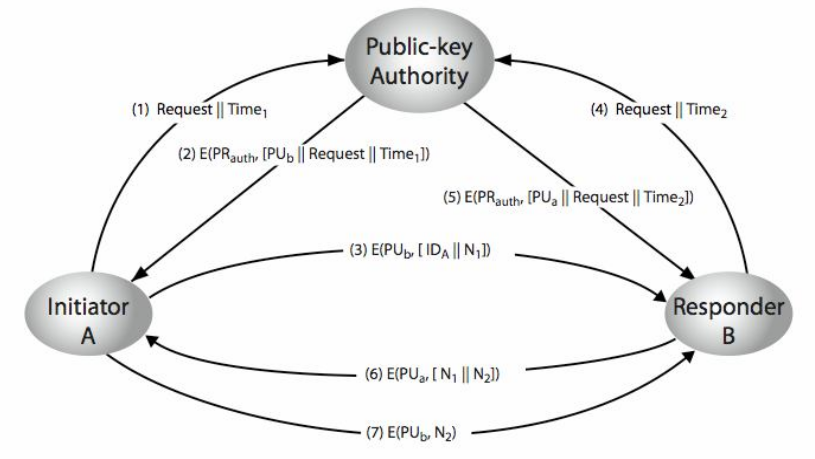Disavantages:

Since the users must appeal to the public-key authority in order to obtain the other users’ public key it can produce a bottleneck.

Public Key Certificates

Another way to exchange keys without the need of a public-key authority is the public-key certificates. The general idea would be:

1. A certificate is a data block that contains a public key plus an identifier of the key’s owner. This data block would be signed by a trusted third party which would be the certificate authority.
2. A user would generate a pair key and send the public key to this certify authority in a secure way and obtain a certificate issued by the certify authority (the trusted third party).
3. This user then would publish this certificate so another user can verify that the certificate was created by the trusted third party.

Please notice that the certificate authority (the trusted third party) is the only one that can create and update certificates.

Steps:

1. User A supply a public key PUa with a request for a certificate to the certificate authority. This request must be done in a secure ways such as in person for example.
2. The certificate authority would provide user A with this from: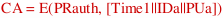where E is the encryption algorithm, PRauth is the authority’s private key and Time1 is a timestamp, and IDa is the user A identification.
3. User A then can pass the certificate CA any user (in this case user B).
4. User B get the certificate from user A and verify if the certificate correspond to the certify authority by decrypting the message using the authority’s public key: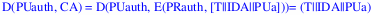In this way it can verify that the certificate is not counterfeit.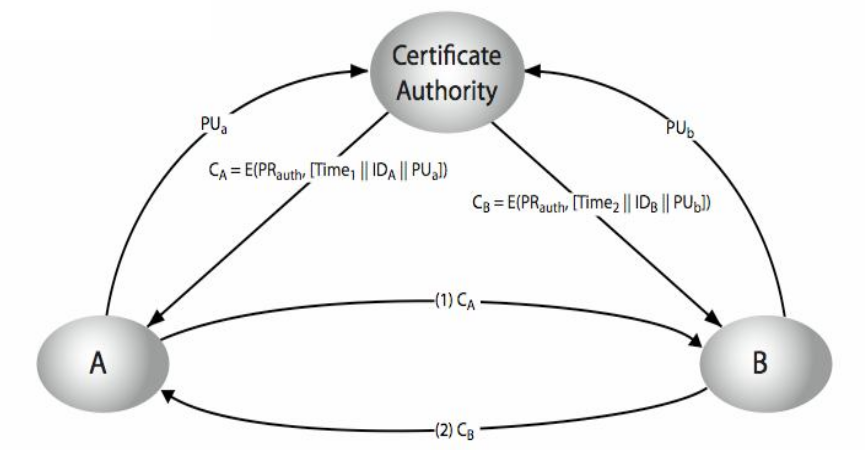## Introduction to Network Security – Part 9

NOTIFICATION: These examples are provided for educational purposes. The use of this code and/or information is under your own responsibility and risk. The information and/or code is given ‘as is’. I do not take responsibilities of how they are used. You are welcome to point out any mistakes in my posting and/or leave a comment.

Some of the mathematical tools (known as number theory) use in network security are prime numbers, greatest common divisor (GCD), Fermat’s theorem, Euler Totient function and theorem, primality testing and Miller Rabin algorithm.

Prime Number

A prime number is an integer p greater than 1 which can only be divided by 1 and itself.

Formally speaking:

“A prime number (or prime integer, often simply called a “prime” for short) is a positive integerthat has no positive integer divisors other than 1 anditself. ” <http://mathworld.wolfram.com/PrimeNumber.html>

An example of prime numbers: 2, 3, 5, 7, 11, 13, 17, 19, 23, 29, 31, 37, …

An example of numbers that are not prime: 1, 4, 6, 8, 9, 10, 12, 14, 15, 16, 18, 20, 21, 22, 24, 25, …

In the website Math.com you can find a prime number calculator that can tell you if a number is prime or not: <http://www.math.com/students/calculators/source/prime-number.htm>

One of the ways we can use prime numbers is to factorize a number in a unique way:

Any number a greater than 1 can be factored in a unique way using prime p numbers while each consecutive prime number is greater than the previous prime number and their consecutive exponents b are one greater that then previous:

a = p1^b1 * p2^b2 * ... * pn^bn while p1 < p2 < ... < pn and (b1, b2, ..., bn) > 0

For example:

1. a = 91 = p1 ^ b1 * p2 ^ b2 = 7 ^ 1 * 13 ^ 1 = 7 * 13
Therefore, P1 = 7 and P2 = 13
2. a = 3600 = p1 ^ b1 * p2 ^ b2 * p3 ^ b3 = 2^4 * 3^2 * 5^2
Therefore, P1 = 2, P2 = 3, and P3 = 5

Greatest Common Divisor (GCD)

The greatest common divisor (gcd) is a number in which you can divide two positive numbers a and b and at the same time is common in a and b.

Formally Speaking:

“The greatest common divisor … of two positive integersandis the largest divisor common toand. ” <http://mathworld.wolfram.com/GreatestCommonDivisor.html>

There are different ways to obtain the greatest common divisor such as using prime factorizations, Euclid’s algorithm, and others <http://en.wikipedia.org/wiki/Greatest_common_divisor>.

Approach 1:

A way to determine the greatest common divisor of two integers a and b is by
comparing the prime factorization of a and b and using their least powers.

Lets assume we wish to obtain the greatest common divisor of 12 and 30, gcd(12, 30).

For 300, we obtain the following prime numbers:

30 = 2^1 * 3^1 * 5^1

For 12, we obtain the following prime numbers:

12 = 2^1 * 3^1 = 2^1 * 3^1 * 5^0

In both cases, we have that 2 and 3 are the prime numbers used in a and b.
Also, we have that 2^1 and 3^1 are their least powers. Therefore,

gcd(12, 30) = 2^1 * 3^1 = 6

Approach 2:

The follow is a more friendly approach to obtain the greates common divisor.
Lets assume we wish to know the greatest common denominator of 7 and 160, gcd(7, 160)

1. Write down this formula:
(Dividend) = (Divisor) * (Quotient) + (Remainder)
2. The greatest number will be the dividend:
Dividend = 160
(160) = (Divisor) * (Quotient) + (Remainder)
3. The other number will be the divisor:
Divisor = 7
(160) = (7) * (Quotient) + (Remainder)
4. Multiply the divisor with a quotient that would get you a number as close as possible to the dividend
If 160/7 = 22.8571429 then use 22 for the quotient
(160) = (7) * (22) + (Remainder)
5. The remainder will be the number that you need to reach 160. Since 7 * 22 is 154 the remainder is 6
(160) = (7) * (22) + (6)
6. Now the Divisor became the new dividend and the remainder became the new divisor:
New dividend = 7 (previous divisor)
New divisor = 6 (previous remainder)
(7) = (6) * (Quotient) + (Remainder)
7. Repeat the process, get a quotient that would multiply the divisor (6) as close as possible to the dividend (7)
(7) = (6) * (1) + (Remainder)
8. The remainder would be 1. This remainder is the greatest common divisor between 7 and 160
gcd(7, 160) = 1

You can find a gcd calculator here:
<http://britton.disted.camosun.bc.ca/gcdlcm/jbgcdlcm.htm>

Fermat’s Theorem

Before going over the Fermat’s theorem, let review an old concept related with this topic, modular arithmetic.

Modular arithmetic (also known as clock arithmetic), is a system in which numbers “wrap around” after reaching a certain value. Of this system, we use the congruence relation on integer known as modulus.

Formally speaking:
“For a positive integer n, two integers a and b are said to be congruent modulo n, (a = b mod n),
if their difference a − b is an integer multiple of n. The number n is called the modulus of the congruence” <http://en.wikipedia.org/wiki/Modular_arithmetic#Congruence_relation>

For Example:

1. Lets assume we have a = 100, b = 86, and n = 7 such that 100 = 86 (mod 7).
2. 100 - 86 = 14 in which 14 has 7 as a divisor.
3. If we divide 100 by 7,
we find out that the quwootient is 14 and remainder is 2.
4. Just coincidence, if we have 100 = 2 (mod 7),
we also find out that the remainder (b) is two too.

Another way to see the previous example is as follows:

100 = 86 (mod 7)

Means that 100 and 86 leave the same remainder when you divide by
7; or, equivalently, that their difference is a multiple of 7.

Here is a link in which you can find the quotient and remainder of a division:
<http://www.analyzemath.com/Calculators_3/quotient_remainder.html>

Another example:

1. For a = 0 mod 5, If a = 0 mod 5 then a^4 = 0^4 = 0 mod 5
2. For a = 1 mod 5, if a = 1 mod 5 then a^4 = 1^4 = 1 mod 5
3. For a = 2 mod 5, if a = 2 mod 5 then a^4 = 2^4 = 16 = 1 mod 5
4. For a = 3 mod 5, if a = 3 mod 5 then a^4 = 3^4 = 81 = 1 mod 5
5. For a = 4 mod 5, if a = 4 mod 5 then a^4 = 4^4 = 256 = 1 mod 5

Now that we have an idea about modulus, we can begin talking about Fermat’s Theorem.

Fermat’s theorem also known as “Fermat’s little theorem” (do not confuse with “Fermat’s last theorem”), establish that:

1. If p is a prime number and for any integer a lower than the prime number p, a<p is a positive integer not divisible by the prime number p.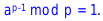2. Or, if p is a prime number then for any integer a, a^p – a will be evenly divisible by p.3. Or, if p is a prime and a is an integer relatively prime to p, then [a^(p−1)] − 1 will be evenly divisible by p.For example:

1. Lets assume p = 3 and a = 2Fermat's little theorem establish that
2.3. Then a^(p-1) = 2^(3 - 1) = 2^2 = 4
4. So, 1 = a^(p-1) mod p = 4 mod 3 = 1

In network security, Fermat’s little theorem is used in public key and primality testing.

Euler Totient Function ø(n)

The Euler Totient function is represented by ø(n) or φ(n) depending the author.

The Euler Totient function establish that:

1. ø(n) is the number of possitive integers less than n and coprime (also known as relatively prime) to n
2. Or the number of positive integers less or equal to n that are relatively prime to n, where 1 is counted as being coprime (relatively prime) to all numbers.
3. Or, ø(n) returns the number of integers less than n (including 1) that are relatively prime to n.

Note: we say that two integers a and b are relatively prime if a and b have no common positive factor other than 1 or, if their greatest common divisor is 1. a is relatively prime to b if gcd(a, b) = 1.

A list of Euler’s Totient Function Values For n = 1 to 500, with divisor lists can be found in the following URL address: <http://primefan.tripod.com/Phi500.html>

For example:

1. Lets n = 37 so ø(n) = ø(37) = 35. 37 can be divided by 1 and by 37.
Therefore, all integers from 1 to 36 are relatively prime to 37
2. Lets n = 35 so ø(n) = ø(35) = 24. 35 can be divided by 1, 5, 7, and 35.
Therefore, integers 1, 2, 1, 2, 3, 4, 6, 8, 9, 11, 12, 13, 16, 17, 18, 19, 22, 23, 24, 26,
27, 29, 31, 32, 33, 34 are relatively prime to 35.

You may ask, I did you found these values (such as ø(35) = 24)?

To find these values:

1. (Case 1) For n = p (a prime), we have that ø(p) = p – 1.
For Example: Lets p = 7 so ø (p) = ø(7), then ø (7) = 7 – 1 = 6.
Therefore ø(7) = 6
2. (Case 2) When n = p^a (power of a prime).
The numbers with common factor with n are: p, 2p, 3p, . . . ,p^(a-1) * p
since there are p^(a-1) of them.
For example: Lets assume we have a prime number p = 5 and a is 2:
ø(p^a) = p^a – p^(a-1) = (p^(a-1)) * (p – 1) = (p^a) * (1 – 1/p)
ø(5^2) = 5^2 – 5^(2 -1) = (5^(2 – 1)) * (5 – 1) = (5^2) * (1 – 1/5)
ø(25) = 5^2 – 5 = 5 * (5 – 1) = (5^2) * (1 – 1/5) = 20
ø(25) = 20 and all integers relative prime to 25 are
1, 2, 3, 4, 6, 7, 8, 9, 11, 12, 13, 14, 16, 17, 18, 19, 21, 22, 23, 24.

As a general case for the Euler’t Totient function ø(n), we have that:

1. Let p be a prime integer dividing n integer so the integers divisible by p are:
p, 2p, 3p, 4p, . . . , (n/p)(p) where there are n/p number of integers.
2. Then, the number of integers not divisible by p are: n – n/p = n * (1 – 1/p)
3. Let q be another prime number dividing n.
If we wish to find the number of integers divisible by neither p or q,
then deduct the n/q multiples of q such that q, 2q, 3q, . . . , (n/q)(q)
4. In case some elements of p and q are common, the n/pq multiples of pq are:
pq, 2pq, 3pq, . . . , (n/pq)(pq) so n/q – n/pq = (n/q)(1- 1/p)
5. Therefore the total of integers not divisible by p or q is:
n(1 – 1/p) – (n/q)(1 – 1/p) = n(1 – 1/p)(1 – 1/q)

General formula for Euler’s Totient Function ø(n):

ø(n) = n * (1 – 1/p1) * (1 – 1/p2) * . . .  * (1 – 1/pm), where p1, p2, . . . , pm are prime factors of n and m is the total number of prime numbers.

Example:

Lets n = 60, p1 = 2, p2 = 4,  p3 = 5 and ø(n= n * (1 – 1/p1) * (1 – 1/p2) * (1 – 1/p3) then

ø( 60) = 60 * (1 – 1/2) * (1 – 1/3) * (1 – 1/5)
ø(60) = (60 – 60/2) * (1 – 1/3) * (1 – 1/5)
ø(60) = (60 – 30) * (1 – 1/3) * (1 – 1/5)
ø(60) = (30) * (1 – 1/3) * (1 – 1/5)
ø(60) = (30 – 30/3) * (1 – 1/5)
ø(60) = (30 – 10) * (1 – 1/5)
ø(60) = (20) * (1 – 1/5)
ø(60) = (20 – 20/5)
ø(60) = (20 – 4)
ø(60) = 16

All integers relative prime to 16 are:
1, 7, 11, 13, 17, 19, 23, 29, 31, 37, 41, 43, 47, 49, 53, 59

Euler’s Totient Function ø(n) using two Prime Numbers:

Let have two prime number p and q such that p ≠ q so ø(pq) = ø(p)*ø(q) = (p-1)*(q-1) in which

1. The set {1, 2, …, pq -1} of integers is less than pq
2. The integers in the set {1, 2, …, pq – 1} are not relatively prime to pq:
{p, 2p, …, (q – 1)p} and {q, 2q, …, (p – 1)q}
3. ø(pq) = (pq – 1) – [(q-1) + (p-1)]
ø(pq) = pq – p – q +1
ø(pq) = (p-1) * (q-1)
ø(pq) = ø(p)*ø(q)

For example:

ø(n) = ø(pq) = (p-1) * (q-1)
ø(21) = ø(3 * 7)
ø(21) = (3 – 1) * (7 – 1)
ø(21) = 2 * 6
ø(21) = 12

Euler’s Theorem

Euler’s Theorem (also known as Fermat-Euler theorem) establish that for every positive integer a relatively prime to n then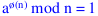For example:

1. Lets a = 3 and n = 10 then
ø(n) = ø(pq) = (p-1) * (q-1)
ø(10)
= ø(2*5) = (2 – 1) * (5 -1) = (1) * (4) = 4
a
^ø(n) mod n = 1
3^ø(10) mod 10 = 1
3^4 mod 10 = 1
81 mod 10 = 1
2. Lets a = 2 and n = 11 then
ø(n) = ø(p – 1)
ø(11)
= ø(11 – 1) = 10
a
^ø(n) mod n = 1
2
^ø(11) mod 11 = 1
2^10 mod 11 = 1
1024 mod 11 = 1

Primality Testing

1. Very large prime numbers selected at random are necessary for most of cryptographic algorithms.
2. Naïve Algorithm: The objective of this algorithm is to divide a number a by all the numbers in turn that are less than the square root of a. This kind of algorithm works for small numbers, but it inefficient for large numbers.

Miller Rabin Algorithm

Miller Rabin algorithm (also known as Miller-Rabin Primality Test) is an algorithm that return a true or false value depending a given value n. Normally, If it outputs true, then n is “probably prime”, else then n is definitely composite.

Approach 1:

[Split Off Power of]: Lets n > 3 and n be odd, k > 0, and  q odd so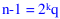1. [Random Base] Choose a random integer a with 1 < a < n.
2. [Odd Power] Set b = a^n (mod m) so if b = ±1 (mod n) then output true and terminate.
3. [Even Powers] For any r with 1 <= r <= k – 1, if b^2^r = -1 (mod n) then output true and terminate else output false

Approach 2:

1. Lets n > 3 and n be odd, k > 0, and  q odd so.
Divide (n – 1) by 2 until the result is an odd number.
2. Let a be an integer 1 < a < n where n > 2 soCheck which of the following two conditions is true:
(a) (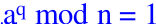) == true ?
(b) There exist 1 <= j <= k such that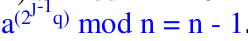== true ?
3. If any of the previous conditions (a and b) are true, then n may not be a prime number.

Example:

1. Lets n = 2047 such that 2047 = 23 * 89.
2. Ifso n – 1 = 2^1 * 1023 then
3. 2^1023 mod 2047 = 1
4. However, 2047 is not a prime

Approach 3: (Similar to approach 1)

1. Check if n integer value is prime or not
2. If n is prime then find integers k > 0, q being odd, such thatis true.
3. ifis true then output is true (n maybe prime) else
4. Check for every value of j going from 1 to k ifis true.
If true then output true (n maybe prime) else
5. return false (n is not prime)

Probabilistic Consideration

For an odd no prime number n and a randomly chosen integer a where 1 < a < n -1,
we can expect a probability of failure in detecting  that n is not a prime number of less than one quarter of the probabiblities.

If we repeat the Millan Rabin algorithm with different values of a, there is a chance that we find a “maybe” prime number n after trying a t number of tests:

Probabilities of finding a “maybe” prime number n after t test are:
Pr(n maybe a prime number after t tests) = (1/4)^t

For example:
Lets assume we wish perform t = 10 tests using different values of a, we have less than 10^-6 probabilities to find an n that maybe a prime number.

Extra Examples

Lets have a = b mod p such that we wish to know a, b = 5^6 and p is 23 so a = 5^6 mod 23

How can we solve this? Using Modulus Arithmetic. One of the properties say that C^(ab) mod p = (C^a mod p)^b mod p

So,

5^6 mod 23 => 5^(2*3) mod 23 => (5^2 mod 23)^3 mod 23 => (25 mod 23)^3 mod 23

The remaider of 25 mod 23 is 2 (23 = 0, 24 = 1, 25 = 2) or you could divide 25 by 23: 23 * 1 = 23 => 25 – 23  = 2 remainer

(25 mod 23)^3 mod 23 => (2)^3 mod 23 => 8 mod 23 = 8 (is less than 23 so is inside the modulus)

## Introduction to Network Security – Part 8

NOTIFICATION: These examples are provided for educational purposes. The use of this code and/or information is under your own responsibility and risk. The information and/or code is given ‘as is’. I do not take responsibilities of how they are used. You are welcome to point out any mistakes in my posting and/or leave a comment.

In security, we use a system of key in order to work on encryption and decryption. The most common system used are the Symmetric Key Encryption and the Public Key Encryption

Symmetric Key Encryption

In a symmetric  system, one key is used for the encryption of a plaintext to a ciphertext and for the decryption of the ciphertext to a plaintext.

The key must be distributed in a secure way to the sender and the receiver making sure that the key is not disclose since then the communication could be compromised. The possible disclose of the key is one of the disadvantages of this system.

Another disadvantages of this system are:

1. There is no way to prove the message was send by the original sender and not from an intruder.
2. The recipient could change the message and say it came from the sender.

Public-key Encryption

In the public key system, normally two keys are generated (pair keys). One key is used to encrypt the message and another key is generated to decrypt the message.

The key that was used for the encryption of the message cannot be used for the decryption and the key used for the decryption of the message cannot be used for the encryption of the plaintext.

One key is the public key which is going to be used for the encryption of the plaintext to the ciphertext and for the verification of the signatures.

The other key is the private key which is going to be used for the decryption of the ciphertext to a plaintext and the generation of signatures.

This system can be used for:

1. Authentication: Verify that the message came from the corresponding sender and the message is received to the corresponding receiver
2. Confidentiality: Create a message that cannot be decrypt by an attacker
3. Authentication and Confidentiality

However, this system still have some main issues such as:

1. Key distribution: In the same way that the symmetric key encryption, there have to be a secure way to distribute keys.
2. Digital Signatures: The way to verify that the message is coming for the sender and not an attacker.

The public-key encryption is considered to be an asymmetric system. This means that those who encrypt the plain-text and/or verify the signatures cannot decrypt the message or create signatures.

In order for a public key encryption to be feasible, it must:

1. Make harder for an attacker to find the key used for the decryption of the ciphertext by just knowing the algorithm and the key used for the encryption of the plaintext.
2. To provide an easy way to decrypt the ciphertext when the key for decryption is used.
3. To provide a way in which either, the private key or public key, can be used for the encryption and the other key used for the decryption of the message. System that implement this policy is called RSA.

This is the way that normally pubic key works:

1. Each user generate a pair of key that will be use for the encryption and decryption.
2. Each user place one key (the public key) to a public register while holding the private key to themselves (the private key is never distributed).
3. In case the private key is change, then the user must generate a new public key that will replace the older public key.

Symmetric Key Encryption Versus Public-Key Encryption

Before we go in deep comparing both encryption systems let clarify some points:

1. The security of both system depend directly on the key/s length. The largest is the key, the harder is to break the cipher.
2. While the public key may provide more security than symmetric key, it produce an overhead. This is the main reason that symmetric key is not considered obsolete with the apparition of the public key encryption.

Here are the differences between symmetric key (conventional) and public key:

1. Symmetric key: Same algorithm using the same key is used for encryption and decrytion.
Public-key: One algorithm is used for encryption and decryption but a pair of keys are generated. One key is used for the encryption, another is used for the decryption.
2. Symmetric key: Sender and receiver must use the same algorithm and share the same key.
Public-key: Sender and receiver must use the same algorithm, but each user must create a pair key. One of those keys (the public key) must be distributed from the receiver to the sender. The other key (private-key), the receiver must kept this key and make sure it doesn’t not get distributed.

Things that need to be resolve from the point of view of security:

1. Symmetric key: The shared key must be kept in secret
Public-key: One of the two keys (normally the private key) must be kept in secret.
2. Symmetric and Public-key: It should be very hard for an attacker to decipher a message if there is no information available.
3. Symmetric key: Even do the attacker may have knowledge of the algorithm and have possession of the ciphertext, it should be very hard to obtain the plaintext and/or the shared key.
Public-key: Even do the attacker may have knowledge of the algorithm, samples of the ciphertext, and the public key, it should be very hard to obtain the plaintext and the other key.

How to Use Public Key Encryption

The public-key encryption can be used to provide:

1. Confidentiality: Prevent attackers to know the content of the message
2. Integrity: Prevent attackers for modifying the original message
3. Authentication: To verify that the sender and/or receiver is not an attacker disguising as the sender and/or receiver
4. Digital Signature:  To verify that the message is send by the sender and not the attacker

Confidentiality (secrecy):

1. For a plaintext X where X = [X1, X2, …, Xn]
2. User A will generate two keys: Public key (PUa) and Private key (PUb)
3. User B will generate two keys: Public key (PUb) and Private key (PUa)
4. For A to send a message to B, A will receive the public key (PUb) from B.
5. User A will encrypt the plaintext (X) using the public key (PUb) from user B with the encryption algorithm (E) to generate the ciphertext (Y).
Y = E(PUb, X)
6. User B will receive the ciphertext (Y). Using private key (PRb) with the decryption algorithm (D), user B will obtain the plaintext (X).
X = D(PRb, Y)

Authentication:

1. User A generate a plaintext for user B. User A encrypt the plaintext (X) using the private key (PRa) and the encryption algorithm (E) then user A send the ciphertext (Y) to user B.
Y = E(PRa, X)
2. User B receive the ciphertext (Y) and using the public key (PUa) with the decryption algorithm (D), user B obtain the plaintext (X).
X = D(PUa, Y)

Even do this provide authentication and provide safety against the alteration of the message, it does not provide confidentiality because:

1. This Authentication do not prevent from eavesdropping.
2. An attacker can decrypt the ciphertext (Y) using user A public key (PUa).

Since tthe message can be prepare only for user A because it was encrypted by using user A’s private key (PUa). this message can be used for the purpose of digital authentication (we can assure the message comes from user A since he provide the public key), and it provide data integrity ( prevention against alteration of the message) since it is impossible to alter the message without the private key (PRa).

Confidentiality and Authentication:

By using the the properties of Confidentiality and Authentication, we can create a scheme that provide more security.

1. User A generates a pair of keys (PUa and PRa) while user B also generates a paid of keys (PUb and PRb)
2. Sending the message: User A uses the private  key (PRa) with the encryption algorithm (E) to encrypt the plaintext (X) to a ciphertext (Y). Then user A uses the public key (PUb) from user B with the encryption algorithm (E) to encrypt the ciphertext again to a new ciphertext (Z).
Z = E(PUb, E(PRa, X))
3. Receiving the message: User B receive the ciphertext (Z) from user A. User B uses the decryption algorithm (D) with the private-key (PRb) with the ciphertext (Z) to produce ciphertext (Y). Then user B uses the public key (PUa) from user A with the decryption algorithm (D) to decrypt the ciphertext (Y) to the plaintext (X).
X = D(PUa, D(PRb, Z))

Requirements for Public Key Encryption

1. It should be easy for user A to generate a pair of keys: Public key (PUa) and private key (PRa).
2. It should be easy for user B to generate a pair of keys: Public key (PUb) and private key (PRb).
3. It should be easy for user A to encrypt the plaintext (M) to a ciphertext (C) using the public key (PUb) from user B.
C = E(PUb, M)
4. It should be easy for user B to decrypt the ciphertext (C) to the plaintext (M) using the private key (PRb).
M = D(PRb, C)
Since C  = E(PUb, M) then M = D(PRb, E(PUb, M))
5. It should be very hard for an attacker while knowing the public key (PUb) from user B to guess correctly the private key (PRb) of user B.
6. It should be very hard for the attacker while knowing the public key (PUb) from user B and the ciphertext (C) encrypted with the public key (PUb) to obtain the plaintext (M) send by user A to user B
7. Both keys should be able to be used in either order for the encryption and decryption:
M = D(PUb, E(PRb, M)) = D(PRb, E(PUb, M))

Algorithm such as RSA follow these requirements.

## Introduction to Network Security – Part 7

NOTIFICATION: These examples are provided for educational purposes. The use of this code and/or information is under your own responsibility and risk. The information and/or code is given ‘as is’. I do not take responsibilities of how they are used.

Transposition Ciphers

The main idea of transposition ciphers is to rearrange the order of the letters used in the plaintext. This prevent the attacker to be able to recognise the message by using the frequency of distributions.

Rail Fence Cipher

Encryption

The basic concept of encryption on Rail Fence cipher is the follow:

1. Select a number of rows greater or equal to two. For this example, we will pick three: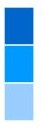2. Place each letter of the message in each row, one letter at a time, on one row at a time, from the top to the bottom
1. Lets assume the plaintext is “SUPERSECRETMESSAGE”
2. Rearrange the letters on the rows: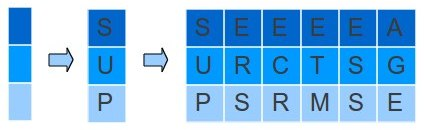3. After finished, we append one row after another in order, forming the ciphertext.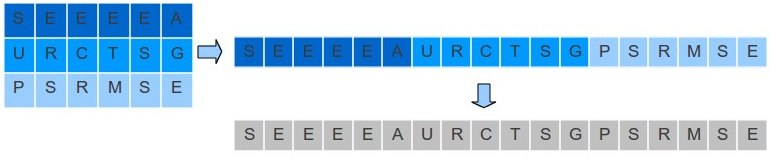Decryption

The decryption of a rail fence cipher is almost the reverse process of the encryption.

1. You will need the ciphertext and the number of rows:
1. The ciphertext is “SEEEEAURCTSGPSRMSE”
2. The number of rows is:
|rows| = 3
2. Computer the length of the ciphertext. In this case, the ciphertext “SEEEEAURCTSGPSRMSE” is:
|ciphertext| = 18
3. Lets calculate the columns that we will have:
Number of Columns = ( |ciphertext| ÷ |rows| ) + ( |ciphertext| mod |rows| )
= ( 18 ÷ 3 ) + (18 mod 3 )
= 6 + 0
= 6 columns
4. Now, we have a table of 3 rows by 6 columns:5. Let fill up this table with the ciphertext, one letter at a time, from top to down and left to right:6. Now recreate the plaintext from this table: